# GMAT Math : DSQ: Calculating compound interest

## Example Questions

### Example Question #1 : Dsq: Calculating Compound Interest

Jenna needs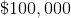years from today to start a business. After talking to an investment banker, Jenna is assured to gain an annually compounded interest of.

What amount of money does Jenna need to invest today?

(1)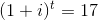(2)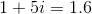Statement (2) ALONE is sufficient, but Statement (1) ALONE is not sufficient

Each statement ALONE is sufficient

Statement (1) ALONE is sufficient, but Statement (2) ALONE is not sufficient

Both statements TOGETHER are sufficient, but NEITHER statement ALONE is sufficient

Both statements TOGETHER are not sufficient.

Statement (2) ALONE is sufficient, but Statement (1) ALONE is not sufficient

Explanation:

Using statement (1), the value of i cannot be determined because t is not specified.

Therefore Statement (1) ALONE is not sufficient.

Using statement (2), the value of i can be determined: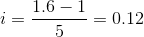We can then set the following equation with X being the amount of money Jenna has to invest today: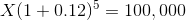We calculate X as follows: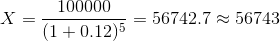### Example Question #2 : Dsq: Calculating Compound Interest

Tyler invests $20,000 in a certificate of deposit that draws compound interest. How frequently is the interest compounded - monthly, bimonthly, or quarterly? Statement 1: The certificate draws 3.15% annual compound interest. Statement 2: The certificate draws$638.33 the first year.

EITHER statement ALONE is sufficient to answer the question.

Statement 2 ALONE is sufficient to answer the question, but Statement 1 ALONE is NOT sufficient to answer the question.

BOTH statements TOGETHER are sufficient to answer the question, but NEITHER statement ALONE is sufficient to answer the question.

BOTH statements TOGETHER are insufficient to answer the question.

Statement 1 ALONE is sufficient to answer the question, but Statement 2 ALONE is NOT sufficient to answer the question.

BOTH statements TOGETHER are sufficient to answer the question, but NEITHER statement ALONE is sufficient to answer the question.

Explanation:

The compound interest equation is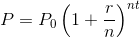whereis the value at the end of the period in question,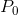is the principal, or initial investment,is the interest rate in decimal form,is the number of times the interest is compounded per year, andis the number of years.

We know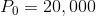from the main body of the problem. It is known that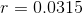only if Statement 1 is knwon, and it is known that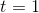only if Statement 2 is known. Also,can only be deduced from Statement 2: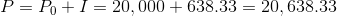Therefore, neither statement alone is sufficient. If both statements are assumed true, the equation becomes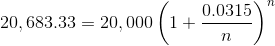or, dividing both sides by 20,000,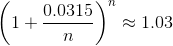The question can be answered by substituting 4 (quarterly), 6 (bimonthly), and 12 (monthly) in turn and seeing which one makes the above statement true.

### Example Question #3 : Dsq: Calculating Compound Interest

Joe invests $4,000 in a certificate of deposit that accrues interest that is compounded monthly. What is the annual interest rate? Statement 1: During the first month, the certificate drew$7.83 in interest.

Statement 2: During the second month, Joe drew $7.85 in interest. Possible Answers: Statement 2 ALONE is sufficient to answer the question, but Statement 1 ALONE is NOT sufficient to answer the question. BOTH statements TOGETHER are insufficient to answer the question. BOTH statements TOGETHER are sufficient to answer the question, but NEITHER statement ALONE is sufficient to answer the question. EITHER statement ALONE is sufficient to answer the question. Statement 1 ALONE is sufficient to answer the question, but Statement 2 ALONE is NOT sufficient to answer the question. Correct answer: EITHER statement ALONE is sufficient to answer the question. Explanation: Assume Statement 1 alone is true. Ifis the annual interest rate, in its decimal form, of a$4,000 CD that draws $7.83 interest in the first month, then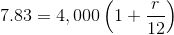This is a linear equation in one variable that can be solved for. Assume Statement 2 is true. Then the equation becomes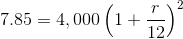This is a quadratic equation in one variable that can be solved for. Either way, the annual interest rate can be found, so either statement alone is sufficient. ### Example Question #4 : Dsq: Calculating Compound Interest Quincy invests$30,000 in a certificate of deposit that draws compound interest. How frequently is the interest compounded - monthly, bimonthly, or quarterly?

Statement 1: The first interest payment is 0.964% of the principal.

Statement 2: The first interest payment is $289.20 Possible Answers: BOTH statements TOGETHER are sufficient to answer the question, but NEITHER statement ALONE is sufficient to answer the question. Statement 2 ALONE is sufficient to answer the question, but Statement 1 ALONE is NOT sufficient to answer the question. Statement 1 ALONE is sufficient to answer the question, but Statement 2 ALONE is NOT sufficient to answer the question. BOTH statements TOGETHER are insufficient to answer the question. EITHER statement ALONE is sufficient to answer the question. Correct answer: BOTH statements TOGETHER are insufficient to answer the question. Explanation: The two statements are equivalent, since 0.964% of$30,000 is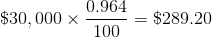Therefore, it can be determined that the interest rate 0.964% is per compounding period. However, no clue is given as to how many compounding periods there are - for example, the annual interest rate is unknown. The two statements together provide insufficient information.

### Example Question #5 : Dsq: Calculating Compound Interest

At the beginning of January of 2015, Olga invested one half of the money she earned in December of 2014 in a certificate of deposit that pays 3.15% annual interest, compounded monthly. At the end of six years, how much will that certificate of deposit be worth?

Statement 1: Olga earned $63,000 in 2014. Stateent 2: Olga earned the same amount of money each month in 2013. Possible Answers: Statement 2 ALONE is sufficient to answer the question, but Statement 1 ALONE is NOT sufficient to answer the question. BOTH statements TOGETHER are sufficient to answer the question, but NEITHER statement ALONE is sufficient to answer the question. BOTH statements TOGETHER are insufficient to answer the question. Statement 1 ALONE is sufficient to answer the question, but Statement 2 ALONE is NOT sufficient to answer the question. EITHER statement ALONE is sufficient to answer the question. Correct answer: BOTH statements TOGETHER are insufficient to answer the question. Explanation: In order to know how much money is in an account after compound interest is accrued, one thing that must be known is the principal, or amount invested. Statement 2 is unhelpful, since information from 2013 is not relevant to information for 2014. From Statement 1 we only know her 2014 earnings, not her December earnings (it is not given that she earned the same per month in 2014). Therefore, the two statements together provide insufficient information. ### Example Question #6 : Dsq: Calculating Compound Interest Natasha decides to invest her entire Christmas bonus in a certificate of deposit that pays 2.15% annual interest, compounded monthly. At the end of five years, how much will that certificate of deposit be worth? Statement 1: Natasha was given a$3,000 Christmas bonus.

Stateent 2: Natasha earns an annual salary of $54,000. Possible Answers: BOTH statements TOGETHER are sufficient to answer the question, but NEITHER statement ALONE is sufficient to answer the question. BOTH statements TOGETHER are insufficient to answer the question. Statement 2 ALONE is sufficient to answer the question, but Statement 1 ALONE is NOT sufficient to answer the question. EITHER statement ALONE is sufficient to answer the question. Statement 1 ALONE is sufficient to answer the question, but Statement 2 ALONE is NOT sufficient to answer the question. Correct answer: Statement 1 ALONE is sufficient to answer the question, but Statement 2 ALONE is NOT sufficient to answer the question. Explanation: In order to know how much money is in an account after compound interest is accrued, the following must be known: The amount of principal, which is given as$3,000 (Natasha's Christmas bonus) only in Statement 1;

The amount of time over which interest is accrued, which is stated to be five years in the main body of the problem;

The frequency with which the interest is compounded, which is given as monthly in the main body of the problem; and,

The rate of interest, which is given as 2.15% in the main body of the problem.

Statement 1 alone provides sufficient information to allow the answer to be calculated. Statement 2 is irrelevant, since it provides no information about Natasha's Christmas bonus.

### Example Question #7 : Dsq: Calculating Compound Interest

Mitchell deposits an amount of money in a savings account that pays compound interest. He does not deposit or withdraw during that time. How much money does he have at the end of five years?

Statement 1: Mitchell deposited $10,000. Statement 2: The savings account draws annual interest of 2.12%, compounded quarterly. Possible Answers: BOTH statements TOGETHER are insufficient to answer the question. Statement 1 ALONE is sufficient to answer the question, but Statement 2 ALONE is NOT sufficient to answer the question. Statement 2 ALONE is sufficient to answer the question, but Statement 1 ALONE is NOT sufficient to answer the question. EITHER statement ALONE is sufficient to answer the question. BOTH statements TOGETHER are sufficient to answer the question, but NEITHER statement ALONE is sufficient to answer the question. Correct answer: BOTH statements TOGETHER are sufficient to answer the question, but NEITHER statement ALONE is sufficient to answer the question. Explanation: In order to know how much money is in an account after compound interest is accrued, the following must be known: The amount of principal, which is given as$10,000 only in Statement 1;

The amount of time over which interest is accrued, which is stated to be five years in the main body of the problem;

The frequency with which the interest is compounded, which is given as quarterly only in Statement 2; and,

The rate of interest, which is given as 2.12% only in Statement 2.

Therefore, both statements together are necessary and sufficient to answer the question.

### Example Question #8 : Dsq: Calculating Compound Interest

John deposits an amount of money in a savings account that pays compound interest. He does not deposit or withdraw during that time. How much money does he have at the end of five years?

Statement 1: John deposited $5,000. Statement 2: The interest is compounded monthly. Possible Answers: Statement 2 ALONE is sufficient to answer the question, but Statement 1 ALONE is NOT sufficient to answer the question. Statement 1 ALONE is sufficient to answer the question, but Statement 2 ALONE is NOT sufficient to answer the question. BOTH statements TOGETHER are insufficient to answer the question. EITHER statement ALONE is sufficient to answer the question. BOTH statements TOGETHER are sufficient to answer the question, but NEITHER statement ALONE is sufficient to answer the question. Correct answer: BOTH statements TOGETHER are insufficient to answer the question. Explanation: Assume both statements are known. In order to know how much money is in an account after compound interest is accrued, the following must be known: The amount of principal, which is stated to be$5,000 in Statement 1;

The amount of time over which interest is accrued, which is stated to be five years in the main body of the problem;

The frequency with which the interest is compounded, which is given to be monthly in Statement 2; and,

The rate of interest, which is not given anywhere.

Insufficient information is given.

Tired of practice problems?

Try live online GMAT prep today.